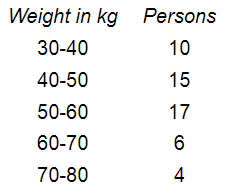# The following is the distribution of weights (in kg) of 52 persons:

Question:

The following is the distribution of weights (in kg) of 52 persons:(i) What is the lower limit of class 50-60?

(ii) Find the class marks of the classes 40-50, 50-60.

(iii) What is the class size?

Solution:

(i) The lower limit of the class $50-60$ is 50 .

(ii) Class mark for the class $40-50$ :

$\frac{40+50}{2}$

$=\frac{90}{2}$

$=45$

Again, class mark for the class $50-60$ :

$\frac{50+60}{2}$

$=\frac{110}{2}$

$=55$

(iii) Here the class size is $40-30$, i.e. 10 .